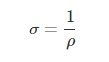# Unit of Conductivity

Conductivity, in general, may be of many types. It could be electrical, ionic, hydraulic, or thermal conductivity. However, conductivity is basically defined as a material’s ability to conduct electricity or heat. Apart from that, measurements are done in several ways and there are specific units of conductivity that are used depending on the circumstances. We will learn about some units below.

## SI Unit of Conductivity

When we talk about electrical conductivity, the SI unit of conductivity is siemens per metre (S/m) or mho and is usually represented by Greek letter sigma, σ, and its formula is given as;As for the SI unit of thermal conductivity, it is measured in watts per metre-kelvin (W/(m K)) or watts per centimetre-kelvin (W/(cm K)). It is represented by the formula;

$$\begin{array}{l}K=\frac{QL}{A\Delta T}\end{array}$$

where,

K is the thermal conductivity in W/m K,

Q is the amount of heat transfer through the material in J/s or W,

A is the area of the body in m2

ΔT is the difference in the temperature in K.

Note: Silver is the most conductive metal followed by copper and gold.

### Other Conductivity Units

Some other units of conductivity also include ohm- metre, BTU, tog, clo, R-value, U-value, ppm, EC, TDS, and CF.

Related articles:

Hope you learned the SI unit of conductivity and other conductivity units. Stay tuned with BYJU’S to know about various science and maths concepts.

## Watch the video and learn how linear expansion varies with the change in temperature.## Frequently Asked Questions – FAQs

Q1

### Define Conductivity.

Conductivity is defined as a material’s ability to conduct electricity or heat.
Q2

### What is the SI unit of Electrical Conductivity?

The SI unit of electrical conductivity is siemens per metre (S/m).
Q3

### What is the SI unit of Thermal Conductivity?

The SI unit of thermal conductivity is watts per metre-kelvin (W/(m K)).
Test your knowledge on Unit Of Conductivity